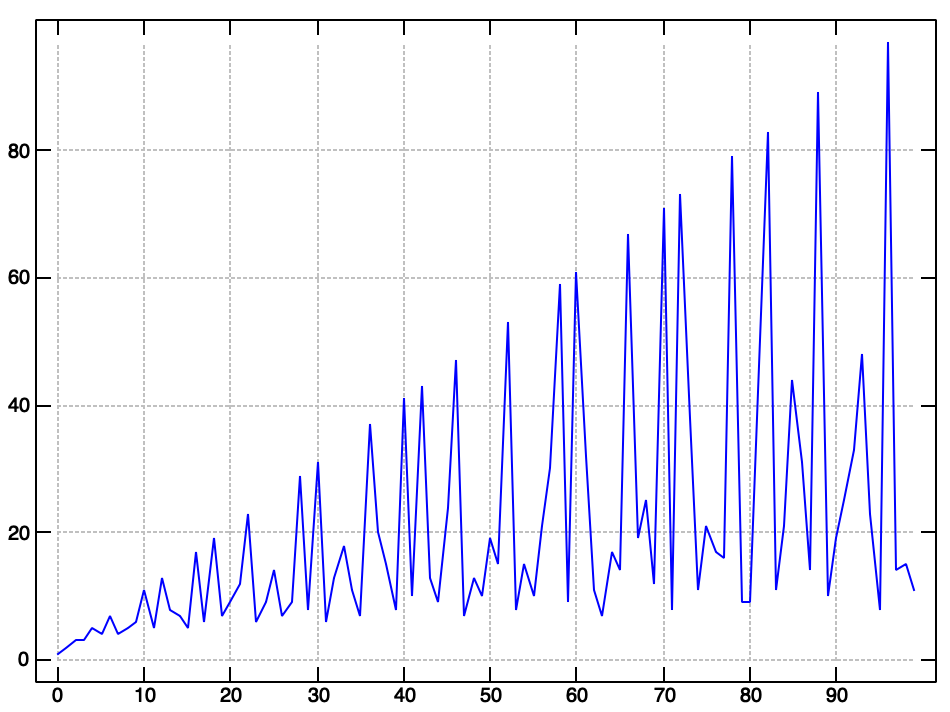I recently watched an interesting 12tone video on the “Euler Gradus Suavitatis” which is a formula devised by Leonhard Euler to analytically compute the consonance, or translated literally, the “degree of pleasure” of a given musical interval.In this formula, `n` can be any positive integer who’s prime factorization is notated as:In reality, `n` can be any ratio of two or more positive integers. Simple intervalic ratios, like an octave, are represented as `1:2`, where `n` would be `2`. For more complicated intervals, like a perfect fifth (`3:2`), we need to find the least common multiple of both components (`6`) before passing it into our Gradus Suavitatis function. We can even do the same for entire chords, like a major triad (`4:5:6`), who’s least common multiple would be `60`.

## The Gradus Suavitatis in J

Intrigued, I decided to implement a version of the Gradus Suavitatis function using the J programming language.

Let’s start by representing our interval as a list of integers. Here we’ll start with a major triad:

``````   4 5 6
4 5 6
``````

Next, we’ll find the least common multiple of our input:

``````   *./ 4 5 6
60
``````

From there, we want to find the prime factors of our least common multiple:

``````   q: *./ 4 5 6
2 2 3 5
``````

Since our primes are duplicated in our factorization, we can assume that their exponent is always `1`. So we’ll subtract one from each prime, and multiply by one (which is a no-op):

``````   _1&+ q: *./ 4 5 6
1 1 2 4
``````

Or even better:

``````   <: q: *./ 4 5 6
1 1 2 4
``````

Finally, we sum the result and add one:

``````   1 + +/ <: q: *./ 4 5 6
9
``````

And we get a Gradus Suavitatis, or “degree of pleasure” of `9` for our major triad. Interestingly, a minor triad (`10:12:15`) gives us the same value:

``````   1 + +/ <: q: *./ 10 12 15
9
``````

## Gradus Suavitatis as a Verb

To make playing with our new Gradus Suavitatis function easier, we should wrap it up into a verb.

One way of building a verb in J is to use the `3 : 0` or `verb define` construct, which lets us write a multi-line verb that accepts `y` and optionally `x` as arguments. We could write our Gradus Suavitatis using `verb define`:

``````   egs =. verb define
1 + +/ <: q: *./ y
)
``````

While this is all well and good, I wasn’t happy that we needed three lines to represent our simple function as a verb.

Another option is to use caps (`[:`) to construct a verb train that effectively does the same thing as our simple chained computation:

``````   egs =. [: 1&+ [: +/ [: <: [: q: *./
egs 4 5 6
9
``````

However the use of so many caps makes this solution feel like we’re using the wrong tool for the job.

Raul on Twitter showed me the light and made me aware of the single-line verb definition syntax, which I somehow managed to skip over while reading the J Primer and J for C Programmers. Using the `verb def` construct, we can write our `egs` verb on a single line using our simple right to left calculation structure:

``````   egs =. verb def '1 + +/ <: q: *./ y'
egs 4 5 6
9
``````

It turns out that there’s over two dozen forms of “entity” construction in the J language. I wish I’d known about these sooner.

Regardless of how we define our verb, the Gradus Suavitatis is an interesting function to play with. Using the `plot` utility, we can plot the “degree of pleasure” of each of the interval ratios from `1:1` to `1:100` and beyond: